Expression with variables

Chapter 11 Class 6 Algebra
Concept wise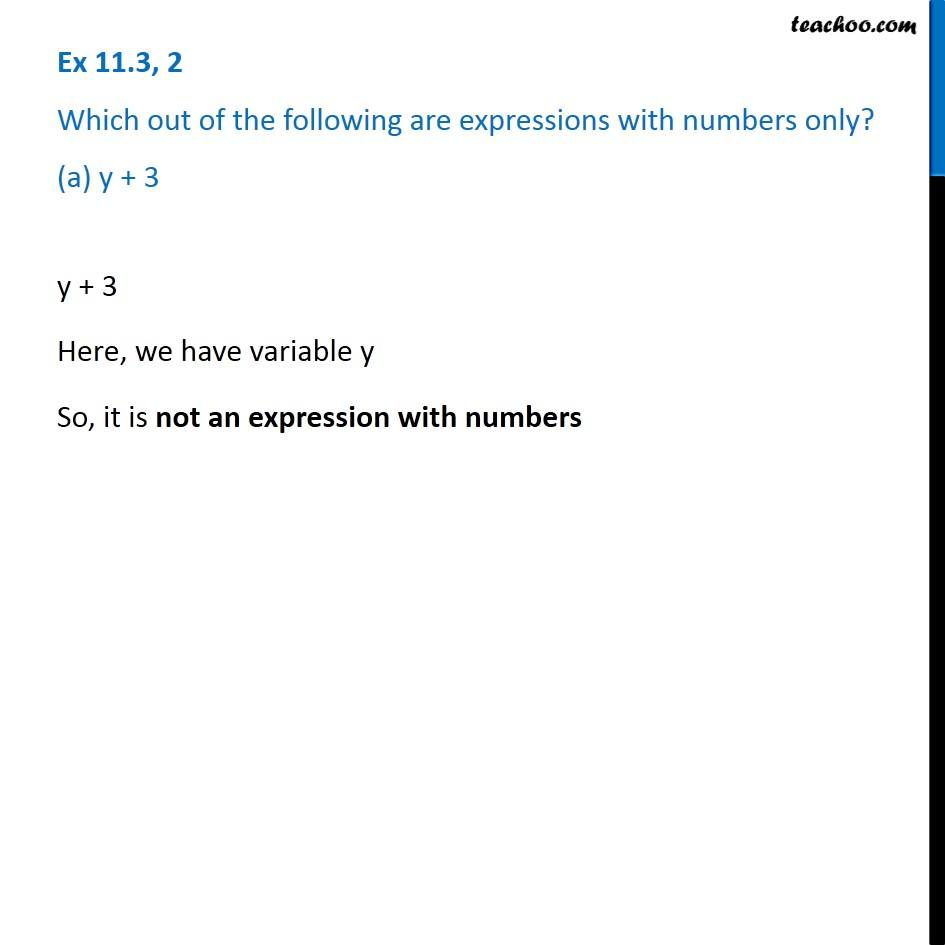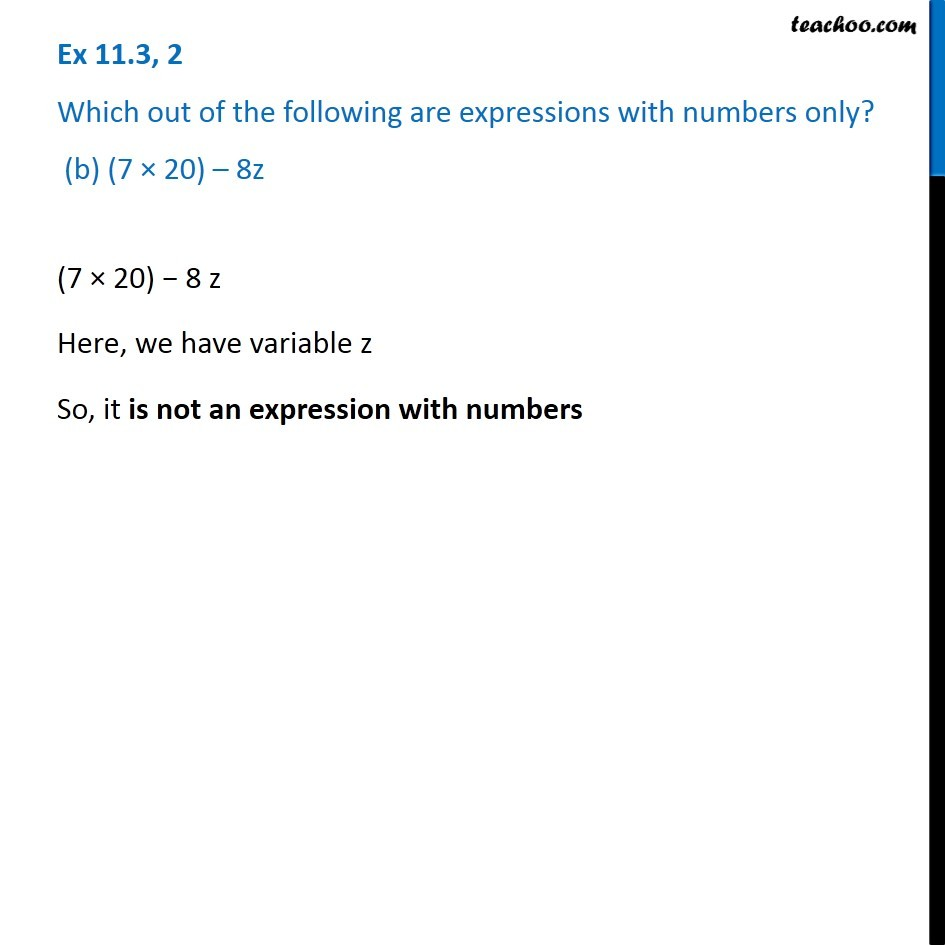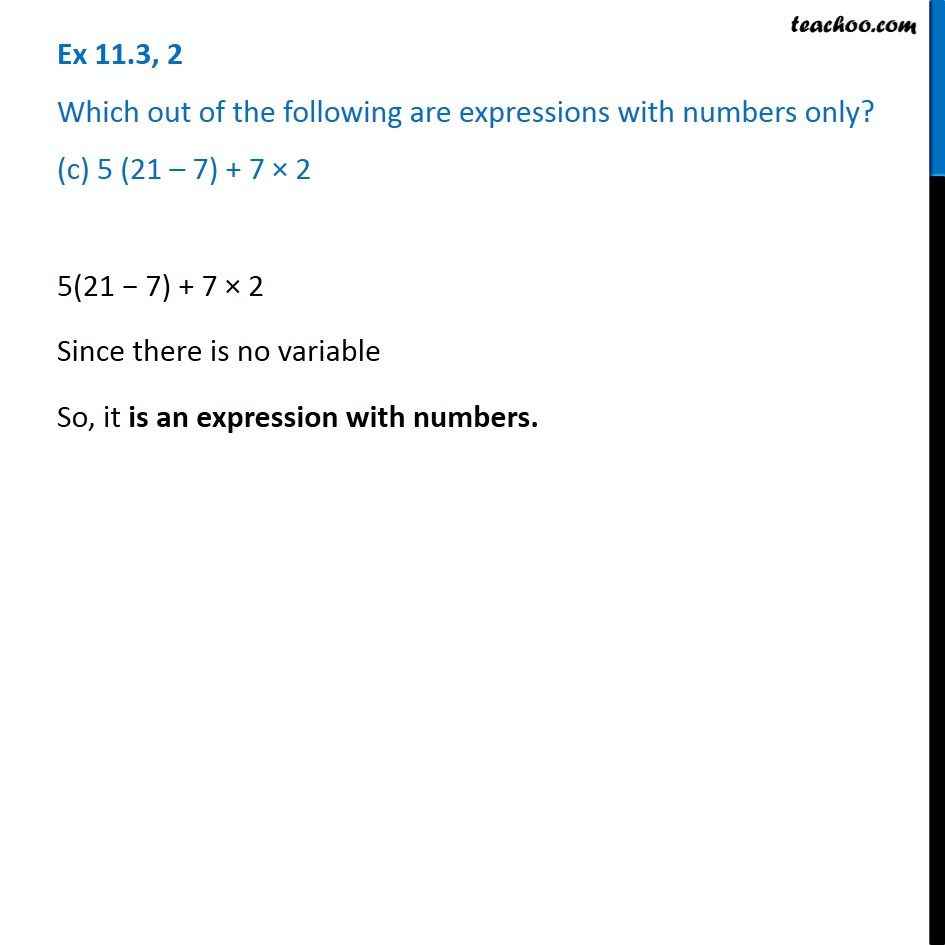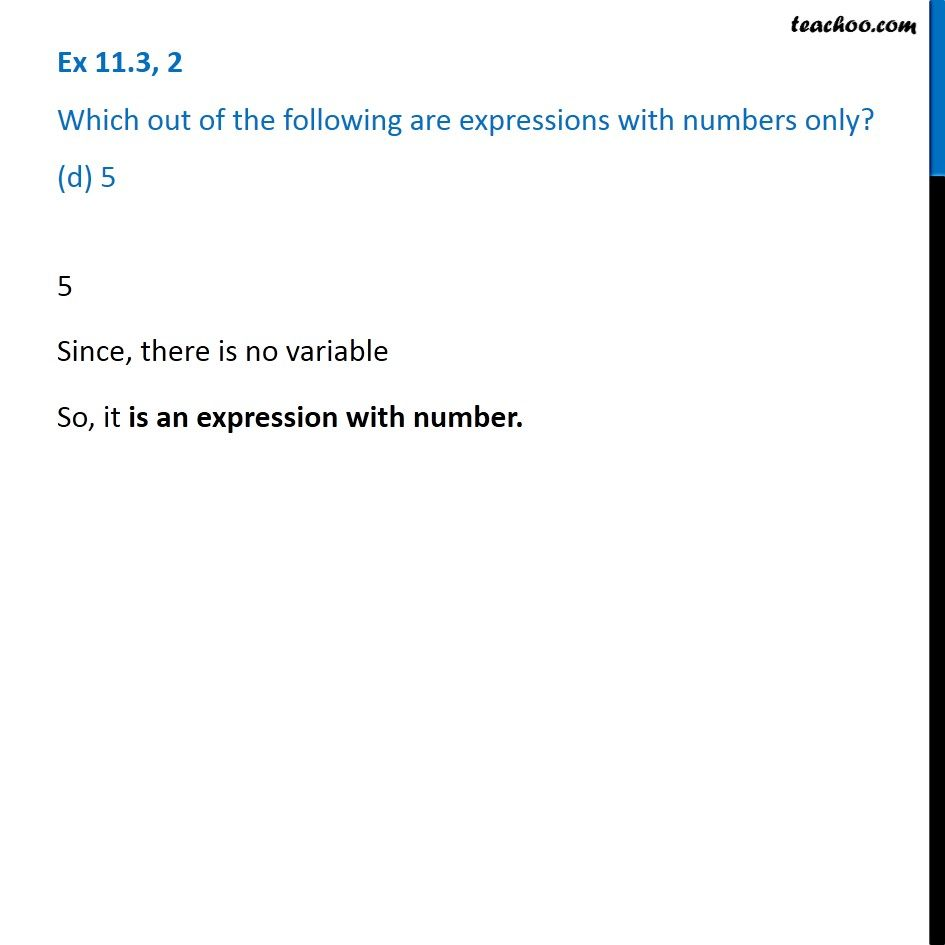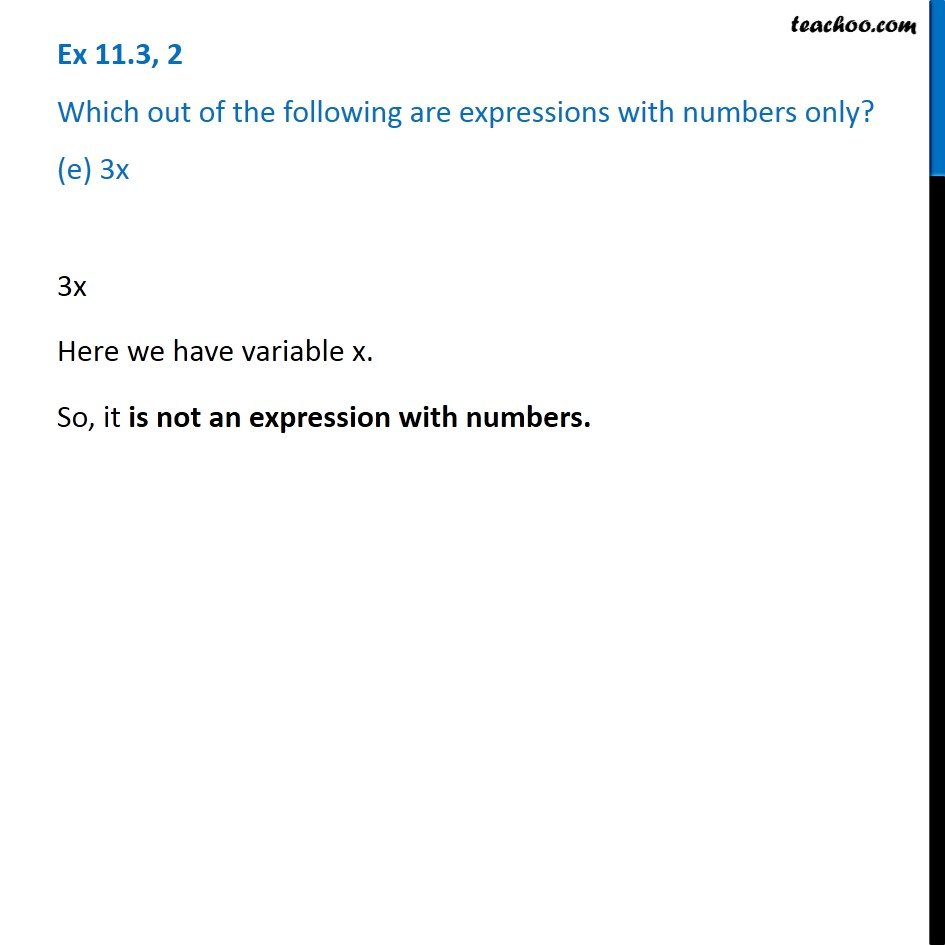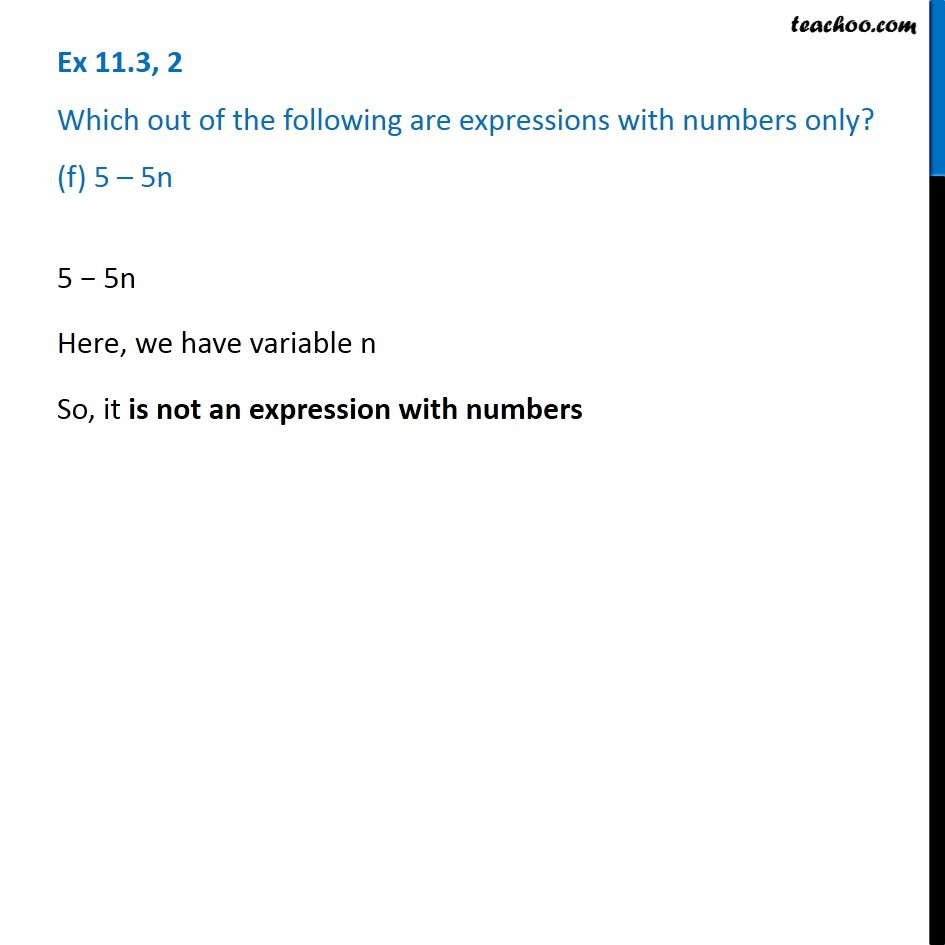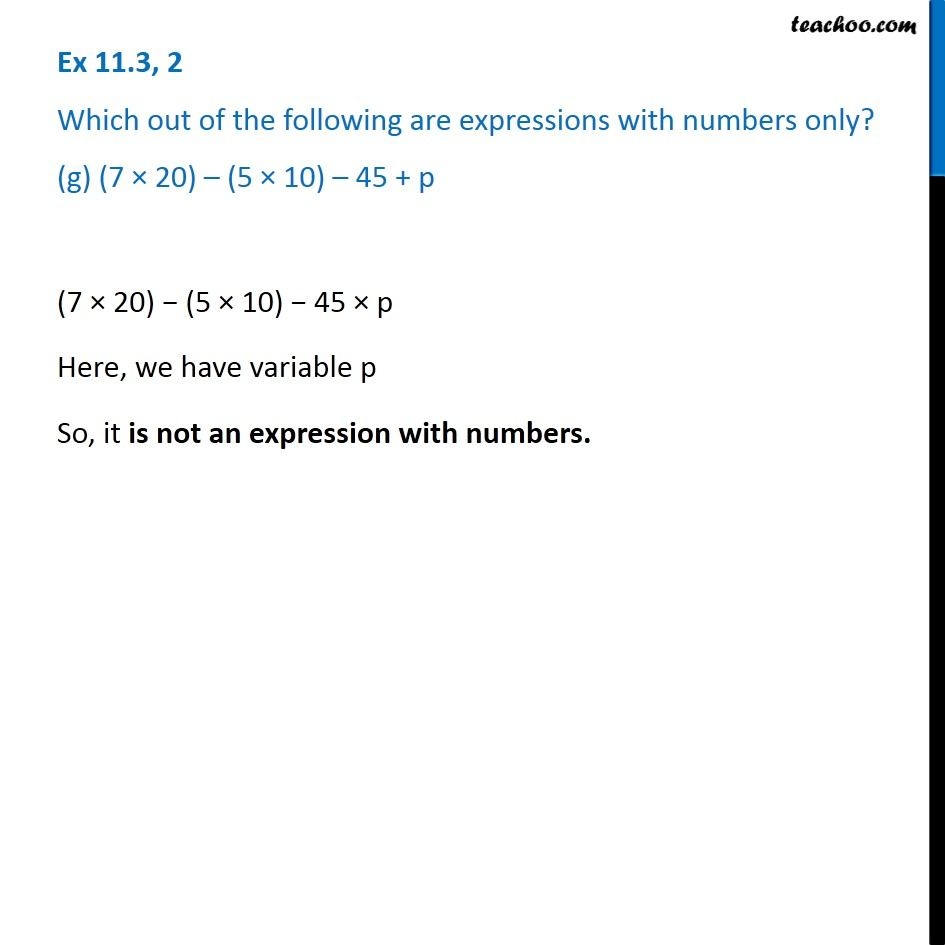Introducing your new favourite teacher - Teachoo Black, at only ₹83 per month

### Transcript

Ex 11.3, 2 Which out of the following are expressions with numbers only? (a) y + 3 Ex 11.3, 2 Which out of the following are expressions with numbers only? (a) y + 3 Ex 11.3, 2 Which out of the following are expressions with numbers only? (a) y + 3 (7 × 20) − 8 z Here, we have variable z So, it is not an expression with numbers Ex 11.3, 2 Which out of the following are expressions with numbers only? (c) 5 (21 – 7) + 7 × 2 5(21 − 7) + 7 × 2 Since there is no variable So, it is an expression with numbers. Ex 11.3, 2 Which out of the following are expressions with numbers only? (d) 5 5 Since, there is no variable So, it is an expression with number. Ex 11.3, 2 Which out of the following are expressions with numbers only? (e) 3x 3x Here we have variable x. So, it is not an expression with numbers. Ex 11.3, 2 Which out of the following are expressions with numbers only? (f) 5 – 5n5 − 5n Here, we have variable n So, it is not an expression with numbers Ex 11.3, 2 Which out of the following are expressions with numbers only? (g) (7 × 20) – (5 × 10) – 45 + p(7 × 20) − (5 × 10) − 45 × p Here, we have variable p So, it is not an expression with numbers.